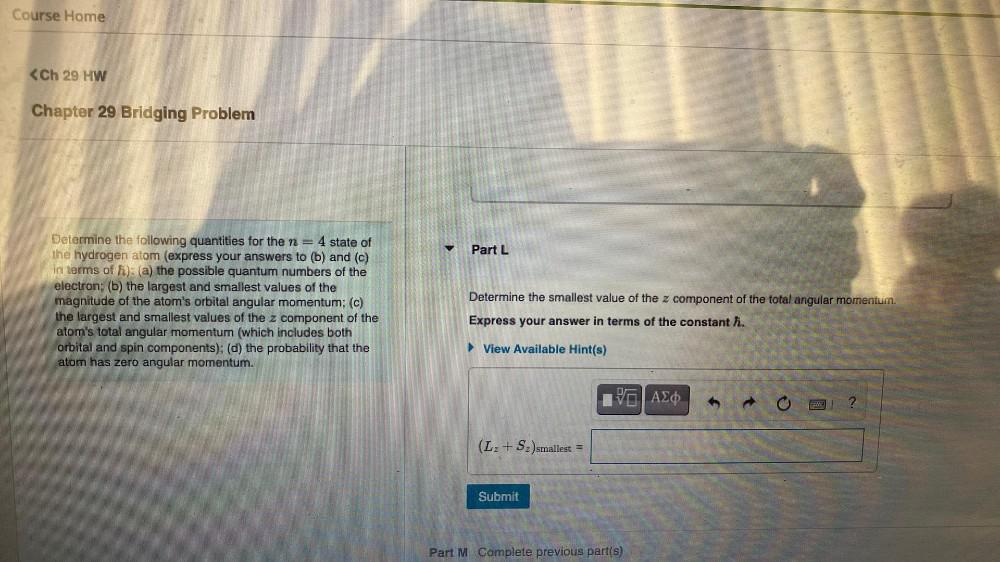Question:

# Course Home <Ch 29 HW Chapter 29 Bridging Problem Part L Determine the following quantities for the n2 = 4 state of the hydrogenCourse Home <Ch 29 HW Chapter 29 Bridging Problem Part L Determine the following quantities for the n2 = 4 state of the hydrogen atom (express your answers to (b) and (c) in terms of h): (a) the possible quantum numbers of the electron; (b) the largest and smallest values of the magnitude of the atom's orbital angular momentum; (c) the largest and smallest values of the z component of the atom's total angular momentum (which includes both orbital and spin components): (d) the probability that the atom has zero angular momentum. Determine the smallest value of the z component of the total angular momentum. Express your answer in terms of the constant h. ► View Available Hint(s) VO AED (L+S-)smallest Submit Part M Complete previous part(s)